## Branch Cuts and Signed Zeros in MATLAB

by on April 28, 2016

Signed zeros are important part of the IEEE754 standard for floating point arithmetic, commonly supported in hardware and in programming languages (C++, C, FORTRAN, etc.).

One of the areas where signed zeros play essential role is correct handling of branch cuts of special functions. In fact, it was one of the reasons why “negative zeros” were introduced in the first place.

The very unpleasant surprise is that MATLAB has poor support for this important feature.

I. Technically it allows user to create negative zeros:

>> x = -0 x = 0   >> 1/x ans = -Inf

The minus sign is not displayed in front of zero (well-known issue of MATLAB) but second operation shows that x is indeed negative.

II. (Real zeros) Sign of real zero is ignored in operations where it plays important role:

% MATLAB/Double precision >> atan(+0-1.5*1i) ans = 1.5707963267949 - 0.80471895621705i >> atan(-0-1.5*1i) ans = 1.5707963267949 - 0.80471895621705i
% MATLAB/Symbolic Math Toolbox (VPA) >> atan(vpa('+0-1.5*i')) ans = -1.570796327 - 0.8047189562 i >> atan(vpa('-0-1.5*i')) ans = -1.570796327 - 0.8047189562 i

In both cases sign of real zero is ignored, and the most amazingly is that different parts of MATLAB do not agree what sign zero has by default (VPA assumes zero is negative, the core function – zero is positive).

## Performance of Elementary Functions

by on April 27, 2016

UPDATE (April 22, 2017): Timings for Mathematica 11.1 have been added to the table, thanks to test script contributed by Bor Plestenjak. I suggest to take a look at his excellent toolbox for multiparameter eigenvalue problems – MultiParEig.

UPDATE (November 17, 2016): All timings in table have been updated to reflect speed improvements in new version of toolbox (4.3.0). Now toolbox computes elementary functions using multi-core parallelism. Also we included timings for the the latest version of MATLAB2016b.

UPDATE (June 1, 2016): Initial version of the post included statement that newest version of MATLAB R2016a uses MAPLE engine for variable precision arithmetic (instead of MuPAD as in previous versions). After more detailed checks we have detected that this is not true. As it turned out, MAPLE 2016 silently replaced VPA functionality of MATLAB during installation. Thus we (without knowing it) tested MAPLE Toolbox for MATLAB instead of MathWorks Symbolic Math Toolbox. We apologize for misinformation. Now post provides correct comparison results with Symbolic Math Toolbox/VPA.

Thanks to Nick Higham, Massimiliano Fasi and Samuel Relton for their help in finding this mistake!

From the very beginning we have been focusing on improving performance of matrix computations, linear algebra, solvers and other high level algorithms (e.g. 3.8.0 release notes).

With time, as speed of advanced algorithms has been increasing, elementary functions started to bubble up in top list of hot-spots more frequently. For example the main bottleneck of the multiquadric collocation method in extended precision was the coefficient-wise power function (.^).

Thus we decided to polish our library for computing elementary functions. Here we present intermediate results of this work and traditional comparison with the latest MATLAB R2016b (Symbolic Math Toolbox/Variable Precision Arithmetic), MAPLE 2016 and Wolfram Mathematica 11.1.0.0.

Timing of logarithmic and power functions in 3.9.4.10481:

>> mp.Digits(34); >> A = mp(rand(2000)-0.5); >> B = mp(rand(2000)-0.5);   >> tic; C = A.^B; toc; Elapsed time is 67.199782 seconds.   >> tic; C = log(A); toc; Elapsed time is 22.570701 seconds.

Speed of the same functions after optimization, in 4.3.0.12057:

>> mp.Digits(34); >> A = mp(rand(2000)-0.5); >> B = mp(rand(2000)-0.5);   >> tic; C = A.^B; toc; % 130 times faster Elapsed time is 0.514553 seconds.   >> tic; C = log(A); toc; % 95 times faster Elapsed time is 0.238416 seconds.

Now toolbox computes 4 millions of logarithms in quadruple precision (including negative arguments) in less than a second!

## MATLAB Error: Cannot load any more object with static TLS

by on February 22, 2016

UPDATE (December 2, 2017): We have found a workaround for the issue and included it into toolbox, this error shouldn’t appear for toolbox versions starting from 4.4.5.

Lately we have been receiving increasing number of reports from users who encounter the following error when trying to use toolbox on GNU Linux version of MATLAB:

>> addpath('/home/user/Documents/AdvanpixMCT-3.9.1.10015'); >> mp.Info Invalid MEX-file '/home/user/Documents/AdvanpixMCT-3.9.1.10015/mpimpl.mexa64': dlopen: cannot load any more object with static TLS   Error in mp.Info (line 196) mpimpl(4);

The error has no relation to our toolbox – it is long standing issue with MATLAB itself, known at least since R2012b release. Overview of the problem can be found on stackoverflow or in our brief explanation below.

Here we focus on how to fix the issue in few simple steps.

## DevNotes #2. Short, Safe and Informative Error Messages from MEX

by on February 14, 2016

MEX API provides two functions for proper handling of erroneous situations: mexErrMsgTxt and mexErrMsgIdAndTxt. Both show error message, interrupt execution of a MEX module and return to MATLAB immediately.

Under the hood the functions are implemented through C++ exceptions. The reason for such design choice is unclear. Throwing C++ exceptions across module boundary (e.g. from dynamic library to host process) is unsafe and generally considered as bad practice in software design. Exceptions are part of C++ run-time library and different versions of it might have incompatible implementations.

## Rational Approximations for the Modified Bessel Function of the Second Kind – K1(x) for Computations with Double Precision

by on January 5, 2016

In this post we will consider minimax rational approximations used for computation of modified Bessel functions of the second kind –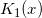. We will follow our usual workflow – we study properties of the existing methods and then propose our original approximations optimized for computations with double precision.

We try to keep the post brief and stick to results. Detailed description of our methodology can be found in previous reports of the series: K0(x), I0(x) and I1(x).

In their pioneering work, Blair & Russon proposed the following approximation forms for:

• For small arguments,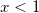:

(1)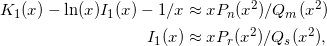• For large values of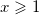:

(2)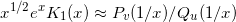All software libraries relying on rational approximations for computinguse (1) and (2) with small variations in coefficients of polynomials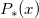and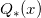.

Relative accuracy shown below is measured in terms of machine epsilon ( eps = 2-52 ˜ 2.22e-16) against “true” values of the function computed in quadruple precision by Maple. Red lines show natural limits for relative error we expect for double precision computations.

Blair & Russon, Boost 1.59.0 and SPECFUN 2.5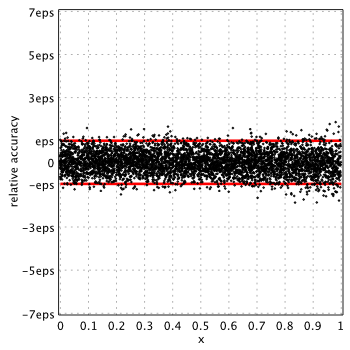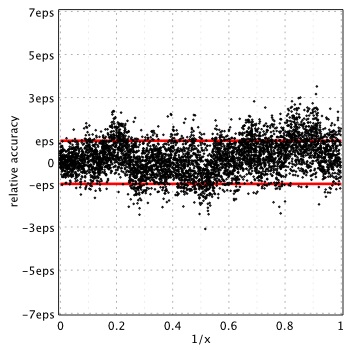Boost and SPECFUN use the same coefficients from original report of Blair & Russon.

## Citations Digest v.5

by on January 5, 2016

Recent papers with references to toolbox:

Books

Papers

Previous issues: digest v.4, digest v.3 and digest v.2.

## Rational Approximations for the Modified Bessel Function of the Second Kind – K0(x) for Computations with Double Precision

by on November 25, 2015

This is the third post in a series of studies on minimax rational approximations used for computation of modified Bessel functions. Previous posts are: I0(x) and I1(x).

Today we will consider modified Bessel function of the second kind –. As usual, at first we check properties of each method and then propose our original approximations which deliver better accuracy.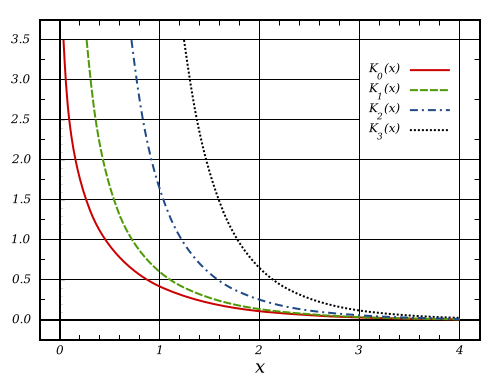Blair & Russon proposed following rational approximations for small arguments,:

(1)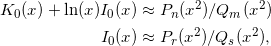For large values ofdifferent function is used:

(2)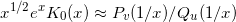## Version 3.9.0 Release Notes

by on November 20, 2015

Over the course of the last three months we have added many important improvements and bug fixes into toolbox. We decided to produce the new official release as it contains critical updates for all platforms (even though eigensolver for large sparse matrices is not included yet).

Here is the main changes:

1. The Core: Multithreading manager has been updated with better load-balancing and non-blocking synchronization. This results in 15-20% performance boost in all operations with dense matrices, even including the matrix multiplication.
2. Eigenvalues.
• Speed of unsymmetric generalized eigen-decomposition has been improved. Now toolbox uses blocked version of Hessenberg tridiagonal reduction which gives us timings lower by 20%.
• Speed of symmetric eigen-decomposition has been boosted up as well. All cases are covered, including the standard and generalized problems in quadruple and arbitrary precision modes and both complexities.

Actual speed-up factor depends on number of cores and matrix size. In our environment Core i7/R2015a/Win7 x64 we see x3 times ratio for 1Kx1K matrix:

mp.Digits(34) A = mp(rand(1000)-0.5); A = A+A';   % Previous versions tic; eig(A); toc; Elapsed time is 48.154 seconds.   % 3.9.0.9919 tic; eig(A); toc; % x3.4 times faster Elapsed time is 14.212 seconds.

Speed-up is even higher for other precision levels:

mp.Digits(50) A = mp(rand(1000)-0.5); A = A+A';   % Previous versions tic; eig(A); toc; Elapsed time is 204.431 seconds.   % 3.9.0.9919 tic; eig(A); toc; % x5.3 times faster Elapsed time is 38.534 seconds.

In fact, all operations with dense symmetric/Hermitian and SPD/HPD matrices have became faster: chol, inv, det, solvers, etc. For example, speed-up ratio for inv is up to x10 times depending on matrix structure and number of cores.

## Rational Approximations for the Modified Bessel Function of the First Kind – I1(x) for Computations with Double Precision

by on November 12, 2015

In connection with our previous study, in this post we analyse existing rational approximations for modified Bessel function of the first kind of order one –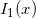.

At first, we consider well known and widely used methods, then propose new approximations which deliver the lowest relative error and have favorable computation structure (pure polynomials).

We study properties of the following rational approximations: Blair & Edwards, Numerical Recipes, SPECFUN 2.5, and Boost 1.59.0. Similar to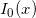, all of them have the structure and intervals, proposed by Blair & Edwards:

(1)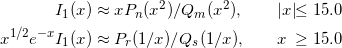whereandare polynomials of corresponding degrees.

Actual accuracy of each approximation in terms of machine epsilon, eps = 2-52 ˜ 2.22e-16, is plotted below:
(Please see our report on I0(x) for technical details).

Blair & Edwards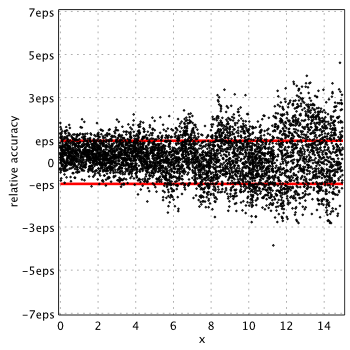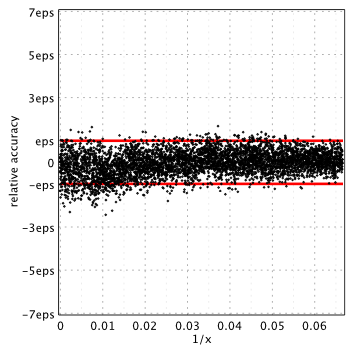## Rational Approximations for the Modified Bessel Function of the First Kind – I0(x) for Computations with Double Precision

by on November 11, 2015

In this post we will study properties of rational approximations for modified Bessel function of the first kindcommonly used to compute the function values in double precision for real arguments. We compare the actual accuracy of each method, discuss ways for improvement and propose new approximation which delivers the lowest relative error.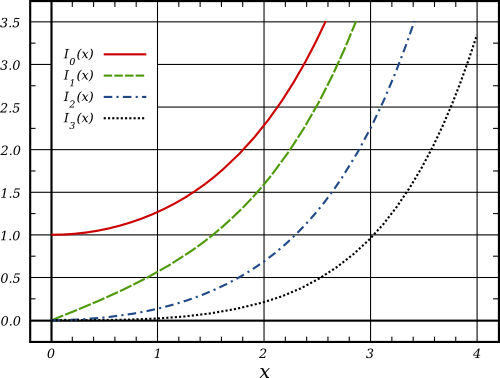We consider following rational approximations: Blair & Edwards, Numerical Recipes, SPECFUN 2.5, and Boost 1.59.0. All libraries use approximation of the same form, proposed in the work of Blair & Edwards:

(1)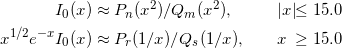whereandare polynomials of corresponding degrees.